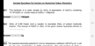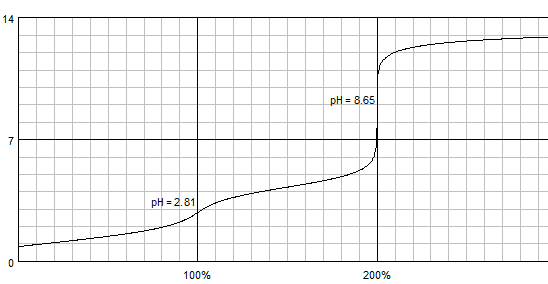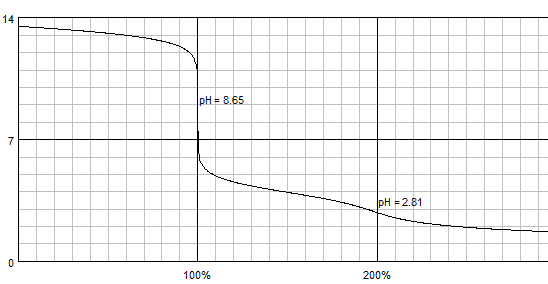# Ionic equilibrium titration

• Chemistry
Prabs3257
Homework Statement:
[" 50mL of "0.5M" Oxalic Acid is needed to neutralize "25mL" of sodium hydroxide "],[" solution.The amount of "NaOH" in "50mL" of the given sodium hydroxide solution is "]
Relevant Equations:
Equation moles
I got a answer by equation the moles i took the n factor of oxalic acid as 2 and the naoh solution came out to be 2M but i got an answer of 4grams but the answer given is 2 grams am i correct ??

#### Attachments

•Screenshot_20191128_193011.jpg
39.7 KB · Views: 167

Homework Helper
It's a bit ambiguous because there are two possible reactions:
OH- + 1/2 H2Ox → H2O + Ox2-
OH- + H2Ox → H2O + HOx-
I've written them that way because you are adding the oxalic acid to the NaOH (by the way, there's no such thing as naoh). You will first form disodium oxalate, then monosodium hydrogen oxalate. The titration will have two endpoints.
Can you estimate the pH at these endpoints? To make it a bit simpler, just try to calculate the pH of a 1M solution of Na2Ox, and a 1M solution of NaHOx. Which do you think best corresponds to "neutralisation" (if that term is not better defined in the problem)?
hint: for oxalic acid, pKa1 = 1.27, pKa2 = 4.27 (This question is assuming a lot of knowledge. How many marks do you get?)

Prabs3257
It's a bit ambiguous because there are two possible reactions:
OH- + 1/2 H2Ox → H2O + Ox2-
OH- + H2Ox → H2O + HOx-
I've written them that way because you are adding the oxalic acid to the NaOH (by the way, there's no such thing as naoh). You will first form disodium oxalate, then monosodium hydrogen oxalate. The titration will have two endpoints.
Can you estimate the pH at these endpoints? To make it a bit simpler, just try to calculate the pH of a 1M solution of Na2Ox, and a 1M solution of NaHOx. Which do you think best corresponds to "neutralisation" (if that term is not better defined in the problem)?
hint: for oxalic acid, pKa1 = 1.27, pKa2 = 4.27 (This question is assuming a lot of knowledge. How many marks do you get?)
this is a high school grade question but why can't we simply equate the milliequivallents of both oxalic acid and NaOH

Homework Helper
Gold Member
Titrations with oxalic acid are most usually done if I remember right by adding alkali to the acid, because you traditionally use as indicator phenolphthalein whose alkaline form is coloured (has pKa about 9) well above the oxalic acid pK's, so both -COOH groups have been titrated when you see the pink colour appear.

I get 4g; the student would have to set out reasoning in more detail for us to identify any misconception or mistake.

Prabs3257
Titrations with oxalic acid are most usually done if I remember right by adding alkali to the acid, because you traditionally use as indicator phenolphthalein whose alkaline form is coloured (has pKa about 9) well above the oxalic acid pK's, so both -COOH groups have been titrated when you see the pink colour appear.

I get 4g; the student would have to set out reasoning in more detail for us to identify any misconception or mistake.
ya i am also getting 4g hence the answer given must be wrong thanks

Homework Helper
Gold Member
What is the source of the question?

Prabs3257
What is the source of the question?
its a sample question for jee mains its an enterance exam in india

Homework Helper
Gold Member
Is 2g the official answer? I don't think I would be able to enter there.
However I hope we shall get a second opinion.

Mentor
Something is definitely wrong, 2 g is amount of NaOH that reacted, not amount of NaOH in 50 mL of the solution.

•epenguin
Homework Helper
Gold Member
Which equivalence point? Are the two of them close-enough for using phenolphthalein as the only indication? My guess is both hydrogens from the oxalic would be strong enough ionized in solution for that one indicator to be good enough; but my judgement could be wrong (unless I review the theory thoroughly).

MentorHomework Helper•